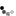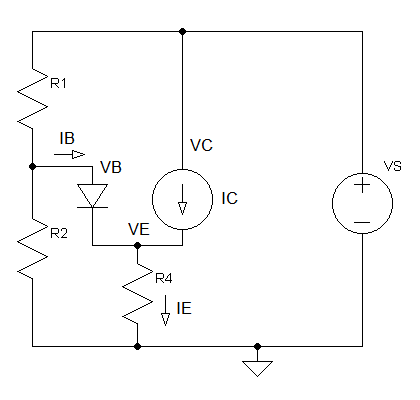# BJT Common Collector Amplifier

A common collector amplifier is one of three basic single-stage bipolar junction transistor (BJT) amplifier configurations. It is also known as an emitter follower and is used as a voltage buffer.

In this circuit the base terminal of the transistor serves as the input, the emitter is the output, and the collector is common to both and connected to the power supply.Notes:
• Measure the simulated results and compare with the theoretical ones by using the formulae derived below. You can obtain the waveform values by clicking within the graph. Assume the following for your calculations
• VBE = 0.7V
• β = 100
• We are using the 2N3904 transistor model. If you construct this circuit in the laboratory correctly using the 2N3904 transistor, you should see similar results.
• Use the Toggle Plot to see the graphs combined or stacked. The stacked plots display maximised waveforms whereas the combined waveforms show their relative values.

## DC Analysis

First we redraw the schematic using the BJT DC model. Capacitors are considered open circuit in DC and therefore are excluded.IB can be ignored if \begin{equation} 10R_2 < \beta R_4 \end{equation} Thus VB can be calculated using KVL as a simple voltage divider circuit \begin{equation} V_B = {R_2 \over {R_1 + R_2}} V_S \end{equation}

Using KVL (Kirchhoff's voltage law) \begin{equation} V_B = I_ER_4 + V_{BE} \end{equation}

To obtain maximum output swing, you should choose the resistor values such that VE is half the supply voltage.

\begin{equation} V_{E} = {V_S \over 2} \end{equation}

## AC Analysis

Next we redraw the schematic using the BJT small signal model. Capacitors are considered shorts in AC and DC supplies are connected to GND (ground). Calculate re

\begin{equation} r_e = {v_T \over I_E} \end{equation}Since the input voltage vi is across re and R4, and using the voltage divider rule, the output voltage is

\begin{equation} v_e = {R_4 \over {r_e + R_4 }}v_i \end{equation}

If re is much smaller than R4, then the voltage gain reduces to unity \begin{equation} {v_e \over v_i} = 1 \end{equation}